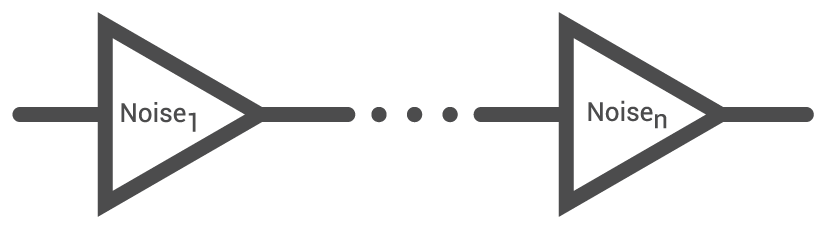# Noise Figure Calculator

## A tool designed to calculate the noise figure of cascaded amplifiers

Noise (dB)
Gain (dB)

(dB) decibel
(dB) decibel

### Overview

Noise figure is the measure of an amplifier's contribution to the overall noise in the system. It is the ratio of the signal-to-noise ratio at the input of the amplifier to the signal-to-noise ratio at the output of the amplifier.

This calculator was designed to help you solve for the noise figure of up to 10 cascaded amplifiers. To use this tool, select the number of cascaded amplifiers and enter the noise and gain (in dB) of each amplifiers. Hitting the "calculate" button will display the total noise figure as well as the overall gain of the cascaded amplifiers.### Equations

$$NF_{total} = 10log_{10}\left(n_{1}+\sum_{M}^{i=2}\frac{n_{i}-1}{\prod_{i-1}^{j=1}g_{j}}\right)$$

$$G_{total} = \sum_{}{}G_{i}$$

Where:

$$n_{i} = 10^{\frac{N_{i}}{10}}$$

$$g_{i} = 10^{\frac{G_{i}}{10}}$$

### Applications

Noise in a circuit is a very important consideration, especially if dealing with extremely weak signals. Noise in electronic communication systems is undesirable but it can only be reduced and not completely eliminated. It is the designer's role to make sure that the noise contribution of each device in the circuit is low enough so that it will not significantly degrade the signal-to-noise ratio.

The total noise figure of a system sets the lower limit on the magnitude of a signal that can be detected in the presence of noise. This means that the best perfomance that a system can achieve is when the noise level is reduced to the lowest possible value. The individual noise figure of a device tells us the noise contribution of that device. For ideal amplifies, the signal-to-noise ratio for both its input and output is infinite, so its noise figure is 1. A real amplifier however will not only amplify the noise at its input but also contribute its own noise to the signal. This means a smaller signal-to-noise ratio at the output of the amplifier and a lower noise figure. Use this tool to calculate the lowest possible noise figure!

Textbook - Amplifiers and Active Devices

Technical Article - Estimating Noise and PSRR in LDOs

Worksheet - Electrical Noise and Interference

1 Comment• A
arike123 April 16, 2016

i think some error in gain calculation . if i know when placing 2 amps in series the total gain in DB is a sum of both amplifiers -    30 db + 30 db = 60 db here in the application the result is 2000?

Like.